IF YOU CAN SEE THIS go to /sfa/Nuprl/Shared/Xindentation_hack_doc.html

### Operator Definitions

An operator definition has the form

<defined op instance> == <expansion>

which stipulates that the left-hand side "expands" to the right-hand side.
Or more generally, for any "instantiation" of the definition, its left-hand side expands to its right-hand side. As a convenience it is also permitted to use function applications on the left-hand-side instead of lambda operators on the right-hand-side; for example the definition of function composition Def (f o g)(x) == f(g(x)) is equivalent to f o g ==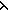x.f(g(x)).

This leaves three things to be explained:
 1 So what if one term expands to another? 2 What is an instance of a definition? 3 What restrictions are there on legitimate definitions?

1. Part of the meaning of an assertion in Nuprl is that it is true iff expanding all the defined operators within it (until none remain) results in a true assertion. Thus, a term can be regarded as meaning whatever it fully expands to.

Below we shall employ the concepts about the form of expressions explained in Terms.

2. Instantiating a definition consists of possible change of binding variables, first- and second-order substitution for free variables throughout the definition, and uniform replacement of op-parm variables by op-parm values or other suitable expressions. See Substitution. Op-parm variables are displayed like "\$x" within terms, except for level-expression variables, which are simply identifiers. Here are examples: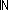expands to {i: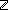| 0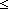i } according to Def== {i:| 0i }

1+2x expands to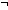x<1+2 according to Def AB ==B<A

x.12 expands to <"x",12> according to Def \$abc.\$n == <"\$abc",\$n>

"Induction for x:. 0<x"
expands to
"0<0 & (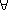x:. 0<x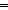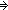0<x+1)(x:. 0<x)"
according to
Def Induction for x:P(x) == P(0) & (x:P(xP(x+1))(x:P(x))

Weird(3; u,vv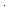v-u) expands to 33-(00-3)
according to
Def Weird(xu,vF(u;v)) == F(F(x;0);x)

HigherConsequence{k'}(0<x) expands to {X:Prop{k''}| 0<xX }
according to
Def HigherConsequence{i}(P) == {X:Prop{i'}| PX }

(Prop{[level]} takes a level-expression; see Prop Levels and Predicativity.)

3. See Op Def Restrictions.

(November 2002 - sfa )